# Power in Tests of Significance

Teaching students the concept of power in tests of significance can be daunting. Happily, the AP Statistics curriculum requires students to understand only the concept of power and what affects it; they are not expected to compute the power of a test of significance against a particular alternate hypothesis.

## What Does Power Mean?

The easiest definition for students to understand is: power is the probability of correctly rejecting the null hypothesis. We’re typically only interested in the power of a test when the null is in fact false. This definition also makes it more clear that power is a conditional probability: the null hypothesis makes a statement about parameter values, but the power of the test is conditional upon what the values of those parameters really are.

To make that even more clear: a hypothesis test begins with a null hypothesis, which usually proposes a very particular value for a parameter or the difference between two parameters (for example, “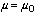” or “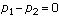”).1 Then it includes “an” alternate hypothesis, which is usually in fact a collection of possible parameter values competing with the one proposed in the null hypothesis (for example, “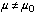” which is really a collection of possible values of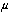, and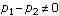," which allows for many possible values of. The power of a hypothesis test is the probability of rejecting the null, but this implicitly depends upon what the value of the parameter or the difference in parameter values really is.

The following tree diagram may help students appreciate the fact that α, β, and power are all conditional probabilities.

### Figure 1: Reality to Decision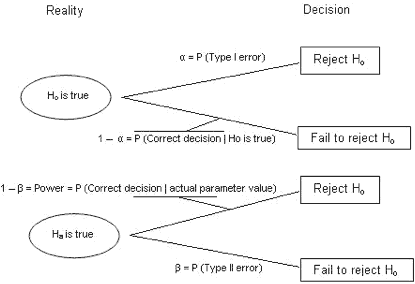Power may be expressed in several different ways, and it might be worthwhile sharing more than one of them with your students, as one definition may “click” with a student where another does not. Here are a few different ways to describe what power is:

• Power is the probability of rejecting the null hypothesis when in fact it is false.
• Power is the probability of making a correct decision (to reject the null hypothesis) when the null hypothesis is false.
• Power is the probability that a test of significance will pick up on an effect that is present.
• Power is the probability that a test of significance will detect a deviation from the null hypothesis, should such a deviation exist.
• Power is the probability of avoiding a Type II error.

To help students better grasp the concept, I continually restate what power means with different language each time. For example, if we are doing a test of significance at level α = 0.1, I might say, “That’s a pretty big alpha level. This test is ready to reject the null at the drop of a hat. Is this a very powerful test?” (Yes, it is. Or at least, it’s more powerful than it would be with a smaller alpha value.) Another example: If a student says that the consequences of a Type II error are very severe, then I may follow up with “So you really want to avoid Type II errors, huh? What does that say about what we require of our test of significance?” (We want a very powerful test.)

## What Affects Power?

There are four things that primarily affect the power of a test of significance. They are:

1. The significance level α of the test. If all other things are held constant, then as α increases, so does the power of the test. This is because a larger α means a larger rejection region for the test and thus a greater probability of rejecting the null hypothesis. That translates to a more powerful test. The price of this increased power is that as α goes up, so does the probability of a Type I error should the null hypothesis in fact be true.
2. The sample size n. As n increases, so does the power of the significance test. This is because a larger sample size narrows the distribution of the test statistic. The hypothesized distribution of the test statistic and the true distribution of the test statistic (should the null hypothesis in fact be false) become more distinct from one another as they become narrower, so it becomes easier to tell whether the observed statistic comes from one distribution or the other. The price paid for this increase in power is the higher cost in time and resources required for collecting more data. There is usually a sort of “point of diminishing returns” up to which it is worth the cost of the data to gain more power, but beyond which the extra power is not worth the price.
3. The inherent variability in the measured response variable. As the variability increases, the power of the test of significance decreases. One way to think of this is that a test of significance is like trying to detect the presence of a “signal,” such as the effect of a treatment, and the inherent variability in the response variable is “noise” that will drown out the signal if it is too great. Researchers can’t completely control the variability in the response variable, but they can sometimes reduce it through especially careful data collection and conscientiously uniform handling of experimental units or subjects. The design of a study may also reduce unexplained variability, and one primary reason for choosing such a design is that it allows for increased power without necessarily having exorbitantly costly sample sizes. For example, a matched-pairs design usually reduces unexplained variability by “subtracting out” some of the variability that individual subjects bring to a study. Researchers may do a preliminary study before conducting a full-blown study intended for publication. There are several reasons for this, but one of the more important ones is so researchers can assess the inherent variability within the populations they are studying. An estimate of that variability allows them to determine the sample size they will require for a future test having a desired power. A test lacking statistical power could easily result in a costly study that produces no significant findings.
4. The difference between the hypothesized value of a parameter and its true value. This is sometimes called the “magnitude of the effect” in the case when the parameter of interest is the difference between parameter values (say, means) for two treatment groups. The larger the effect, the more powerful the test is. This is because when the effect is large, the true distribution of the test statistic is far from its hypothesized distribution, so the two distributions are distinct, and it’s easy to tell which one an observation came from. The intuitive idea is simply that it’s easier to detect a large effect than a small one. This principle has two consequences that students should understand, and that are essentially two sides of the same coin. On the one hand, it’s important to understand that a subtle but important effect (say, a modest increase in the life-saving ability of a hypertension treatment) may be demonstrable but could require a powerful test with a large sample size to produce statistical significance. On the other hand, a small, unimportant effect may be demonstrated with a high degree of statistical significance if the sample size is large enough. Because of this, too much power can almost be a bad thing, at least so long as many people continue to misunderstand the meaning of statistical significance. For your students to appreciate this aspect of power, they must understand that statistical significance is a measure of the strength of evidence of the presence of an effect. It is not a measure of the magnitude of the effect. For that, statisticians would construct a confidence interval.

## Two Classroom Activities

The two activities described below are similar in nature. The first one relates power to the “magnitude of the effect,” by which I mean here the discrepancy between the (null) hypothesized value of a parameter and its actual value.2 The second one relates power to sample size. Both are described for classes of about 20 students, but you can modify them as needed for smaller or larger classes or for classes in which you have fewer resources available. Both of these activities involve tests of significance on a single population proportion, but the principles are true for nearly all tests of significance.

### Activity 1: Relating Power to the Magnitude of the Effect

In advance of the class, you should prepare 21 bags of poker chips or some other token that comes in more than one color. Each of the bags should have a different number of blue chips in it, ranging from 0 out of 200 to 200 out of 200, by 10s. These bags represent populations with different proportions; label them by the proportion of blue chips in the bag: 0 percent, 5 percent, 10 percent,... , 95 percent, 100 percent. Distribute one bag to each student. Then instruct them to shake their bags well and draw 20 chips at random. Have them count the number of blue chips out of the 20 that they observe in their sample and then perform a test of significance whose null hypothesis is that the bag contains 50 percent blue chips and whose alternate hypothesis is that it does not. They should use a significance level of α = 0.10. It’s fine if they use technology to do the computations in the test.

They are to record whether they rejected the null hypothesis or not, then replace the tokens, shake the bag, and repeat the simulation a total of 25 times. When they are done, they should compute what proportion of their simulations resulted in a rejection of the null hypothesis.

Meanwhile, draw on the board a pair of axes. Label the horizontal axis “Actual Population Proportion” and the vertical axis “Fraction of Tests That Rejected.”

When they and you are done, students should come to the board and draw a point on the graph corresponding to the proportion of blue tokens in their bag and the proportion of their simulations that resulted in a rejection. The resulting graph is an approximation of a “power curve,” for power is precisely the probability of rejecting the null hypothesis.

Figure 2 is an example of what the plot might look like. The lesson from this activity is that the power is affected by the magnitude of the difference between the hypothesized parameter value and its true value. Bigger discrepancies are easier to detect than smaller ones.

### Figure 2: Power Curve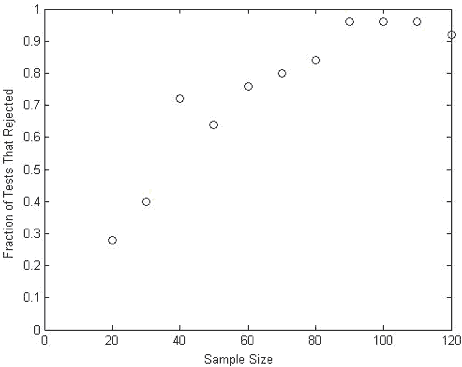### Activity 2: Relating Power to Sample Size

For this activity, prepare 11 paper bags, each containing 780 blue chips (65 percent) and 420 nonblue chips (35 percent).3 This activity requires 8,580 blue chips and 4,620 nonblue chips.

Pair up the students. Assign each student pair a sample size from 20 to 120.

The activity proceeds as did the last one. Students are to take 25 samples corresponding to their sample size, recording what proportion of those samples lead to a rejection of the null hypothesis p = 0.5 compared to a two-sided alternative, at a significance level of 0.10. While they’re sampling, you make axes on the board labeled “Sample Size” and “Fraction of Tests That Rejected.” The students put points on the board as they complete their simulations. The resulting graph is a “power curve” relating power to sample size. Below is an example of what the plot might look like. It should show clearly that when p = 0.65 , the null hypothesis of p = 0.50 is rejected with a higher probability when the sample size is larger.

(If you do both of these activities with students, it might be worth pointing out to them that the point on the first graph corresponding to the population proportion p = 0.65 was estimating the same power as the point on the second graph corresponding to the sample size n = 20.)

## Conclusion

The AP Statistics curriculum is designed primarily to help students understand statistical concepts and become critical consumers of information. Being able to perform statistical computations is of, at most, secondary importance and for some topics, such as power, is not expected of students at all. Students should know what power means and what affects the power of a test of significance. The activities described above can help students understand power better. If you teach a 50-minute class, you should spend one or at most two class days teaching power to your students. Don’t get bogged down with calculations. They’re important for statisticians, but they’re best left for a later course.

### Notes

1. Of the hypothesis tests in the AP statistics curriculum, of which only the chi-square tests do not involve a null that makes a statement about one or two parameters. For the rest of this article, I write as though the null hypothesis were a statement about one or two parameter values, such as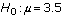or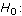2. In the context of an experiment in which one of two groups is a control group and the other receives a treatment, then “magnitude of the effect” is an apt phrase, as it quite literally expresses how big an impact the treatment has on the response variable. But here I use the term more generally for other contexts as well.
3. I know that’s a lot of chips. The reason this activity requires so many chips is that it is a good idea to adhere to the so-called “10 percent rule of thumb,” which says that the standard error formula for proportions is approximately correct so long as your sample is less than 10 percent of the population. The largest sample size in this activity is 120, which requires 1,200 chips for that student’s bag. With smaller sample sizes you could get away with fewer chips and still adhere to the 10 percent rule, but it’s important in this activity for students to understand that they are all essentially sampling from the same population. If they perceive that some bags contain many fewer chips than others, you may end up in a discussion you don’t want to have, about the fact that only the proportion is what’s important, not the population size. It’s probably easier to just bite the bullet and prepare bags with a lot of chips in them.

### Authored by

Floyd Bullard
North Carolina School of Science and Mathematics
Durham, North Carolina